## Look at small cases

### Quick description

A standard question of Gel'fond's, when listening to a talk, is: "What is the simplest nontrivial example?" This article shows how useful this principle can be.

### Example 1: Counting and the Lagrange Identity

This example is borrowed from this blog post. It is a prime example of how a proof for a general argument can be worked out by considering simple cases. The Lagrange identity for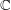says that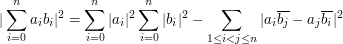(1)

We will analyze the case for n=3. It will provide a beautiful outline for a general proof.

The left hand side of the equality (1) will be equal to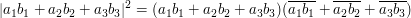(2)

It would be helpful indeed to consider the following 3 x 3 matrix for the sum of all the elements of this matrix is equal to the LHS of (2).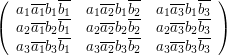In fact, the elements of the matrix are the terms on the RHS in (2).

Each term of the matrix above can thus be represented as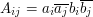Now consider the first term on the RHS in (1). For n=3, it is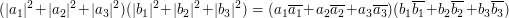(3)

Again, as above, it would be helpful to consider the following matrix.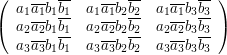The elements of this matrix are again the terms on RHS in (3).

We can thus call each of them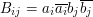The last term in (1) is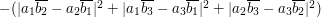(4)

This time we use two matrices to organize the terms in (4).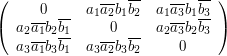and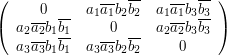And we thus define elements of the first and the second matrix as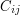and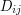respectively where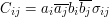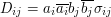and where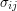is defined to beif i=j and is equal to unity otherwise.

Now let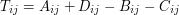Therefore,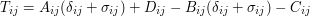where we have used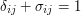(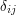is the Kronecker delta)

And so,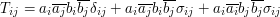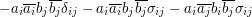or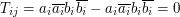Since each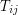is zero, the sum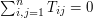which automatically implies the Lagrange Identity in.

This article should not be a stub. It is infact the best suggestion I have come across here. To see how it can lead to some 'mathematically interesting information',I suggest this webpage- http://disquisitionesmathematicae.wordpress.com

### I marked it as a stub because

I marked it as a stub because it had neither a "General discussion" section nor any examples. It is certainly interesting and valuable advice, but doesn't quite count as "mathematically interesting information" in the intended sense, because it doesn't yet contain any actual mathematics. But if, say, you were to add the example that is explained on the page you suggest, then it would instantly lose its stub status.

Just to repeat, it is possible for a Tricki article to contain something interesting, but still to count as a stub. So you should not take the stub designation as in any way suggesting that Gel'fond's suggestion is uninteresting. But I would see the quotation as the beginning of what should ultimately be a quite long article with several examples: a principle this important deserves nothing less.

### Absolutely.There should be a

Absolutely.There should be a string of articles.Hopefully, many more articles would come up with an explanation.Tricky is going to be a great ride.

### I have added the example

I have added the example mentioned above. It might still require some editing though. You may state some prerequisites for the above example but I personally think that it's fairly straightforward and that elementary linear algebra would suffice.

The following comments were made inline in the article. You can click on 'view commented text' to see precisely where they were made.

### I know I suggested putting

I know I suggested putting this example here, but now I look at it carefully, I wonder whether it really is a prime example of Gel'fond's advice. It seems to me that the basic proof (even if you dress it up with matrices) is this: expand out both sides and observe that you get the same answer. And it's not clear to me that doing that for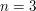is any easier than doing it for the general case.

A truly convincing example of this trick is one where the only realistic way of doing the general case is to do small cases and spot a non-obvious pattern.

### Maybe you are right. There

Maybe you are right. There are loads of problems that can be solved by doing specific cases only. Lucas's identity in elementary number theory might be a good example.(Adding the terms in the Pascal's triangle diagonally yields the Fibonacci numbers)

### I just noticed that there is

I just noticed that there is a topic in 'What kind of problem are you trying to solve' titled 'Prove the result for some cases and deduce it for the rest'. It would be nice if we could link that topic to this article in some way.

### I think there is an important

I think there is an important distinction between the idea of that article and the idea of this. It's the distinction between proving a result for a few simple cases and deducing the rest, and proving it in a few special cases in order to get ideas about how to prove the general result. I think putting a link might blur this distinction in an unhelpful way.

## Post new comment

(Note: commenting is not possible on this snapshot.)

Before posting from this form, please consider whether it would be more appropriate to make an inline comment using the Turn commenting on link near the bottom of the window. (Simply click the link, move the cursor over the article, and click on the piece of text on which you want to comment.)